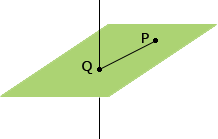Subject: in 3d space Name: marie Who are you: Student find the distance from the point (1, -2, -3) to the line x = y = z-2 Hi Marie, Use the point p(1, -2, -3) and the equation of the line to find the equation of the plane through P and normal to the line. Find the point Q where the plane and the line intersect.The line segment PQ is then normal to the line and hence Q is the closest point on the line to P. Thus the length of the line segment PQ is the distance from P to Q. Penny